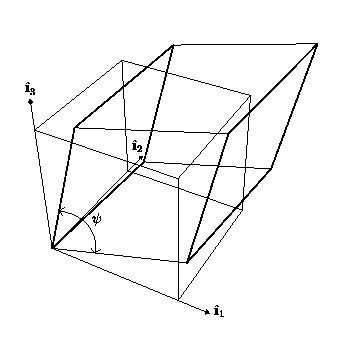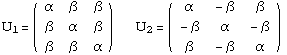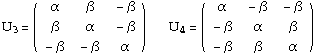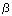## Cubic-to-Trigonal Transition

#### Introduction

The cubic-to-trigonal transition is described by two parameters, a trigonal angleand a stretch. The trigonal angle is the angle between two intersecting edges of the parallelepiped forming a trigonal unit cell; while, the stretch is proportional to the ratio of the length of a side of the cubic unit cell and the length of a side of the trigonal unit cell. The trigonal angle is, however, the fundamental parameter describing the transformation, because for all alloys considered, the stretch is nearly unity.

Some alloys undergoing this transition are

• Au-Cd, Terfenol (Tb-Dy-Fe), Ti-Ni, Ti-Ni-Al, Ti-Ni-Fe.
In Ti-Ni, this is the R-phase; R denotes rhombohedral which is equivalent to trigonal.

There are several possible transitions between a cubic Bravais lattice and a trigonal Bravais lattice:

1. the transition between simple cubic and trigonal is evident, and
2. the transition from either body-centered cubic (BCC) or face-centered cubic (FCC) to trigonal follows from the well-known fact that each of these cubic lattices contains a trigonal unit cell.
Figure 1 shows a cubic unit cell and a trigonal unit cell along with the trigonal angle.Figure 1: Trigonal unit cell (dark thick lines) and a cubic unit cell.

Notice, that for trigonal angles less than 90 degrees, the transformation can be achieved by an expansion along the body diagonal  of the cube; while, for trigonal angles greater than 90 degrees, it is a contraction along the body diagonal  of the cube which gives a trigonal unit cell.

#### Transformation Stretch Matrices

The transformation stretch matrices are the gradient of the deformations which take a cubic lattice into a trigonal lattice. There are 4 such matrices, one for each variant of the martensite phase. They have componentsThe componentsandare functions of the trigonal angle. Specific details can be found in reference . Also, James and Kinderlehrer  have studied some microstructures in this transition during their study of magnetostriction in Terfenol.

#### Microstructures for the Cubic-to-Trigonal Transition

Here are links to various pages, where a number of different microstructures are discussed. Also contained therein is a table showing the possible microstructures for the cubic-to-trigonal transition.

#### References

1. ``Microstructure in the cubic to trigonal transition.'' by Kevin F. Hane and Thomas W. Shield. To be submitted to Materials Science and Engineering A, 1998.

2. ``Theory of magnetostriction with applications to Tbx Dy1-x Fe2.'' by Richard D. James and David Kinderlehrer, Philosophical Magazine B 68, 237 (1993).